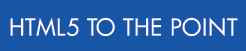# Creating canvas images

You can use the canvas element to create a drawing area (the "canvas"). After you create a canvas, you can draw graphics inside the canvas using JavaScript.

You can write scripts to draw graphics inside a canvas element, but you should use an application to draw anything more than basic shapes. For example, you can use Flash CS5 to create complex and animated graphics for a canvas element using JavaScript.

See www.canvasdemos.com for examples and development tools. For a full tutorial on using all of the canvas element's methods, see developer.mozilla.org/en/Canvas_tutorial.

The following table explains the functions that can be used to draw basic shapes.

Shape

Functions

Description

arcs

arc(x, y, radius, startAngle, endAngle, anticlockwise)

startAngle – the start point of the arc (in radians)
endAngle – the end point of the arc (in radians)
anticlockwise – Boolean value to draw the arc anticlockwise

You can use the following expression to convert degrees to radians:
var radians = degrees * (Math.PI/180)

curves – Bézier

bezierCurveTo(cp1x, cp1y, cp2x, cp2y, x, y)

cp1x – the x coordinate of the first control point
cp1y – the y coordinate of the first control point
cp2x – the x coordinate of the second control point
cp2y – the y coordinate of the second control point

cp1x – the x coordinate of the first control point
cp1y – the y coordinate of the first control point

lines / paths

beginPath()
closePath()
fill()
lineTo(x,y)
moveTo(x,y)
stroke()

Starts a new shape
Draws a line to the start point of the first path
Fills the shape
Draws a line starting at the previous point
Starts a new line
Draws an outline shape

rectangles

fillRect(x,y,width,height);
strokeRect(x,y,width,height);
clearRect(x,y,width,height);

Draws a filled rectangle
Draws a rectangular outline
Makes the specified area transparent

rectangles

rect(x, y, width, height)

x and y – the top left corner's location

## Code

<script>
function draw() {
var canvas = document.getElementById('myShape');
if (canvas.getContext) {
var drw = canvas.getContext('2d');
"rgb(0,100,0)";
drw.fillRect(10,10,100,50);
} }
</script>

<canvas id="myShape" width="300" height="300">A green rectangle</canvas>

## Example

A green rectangle